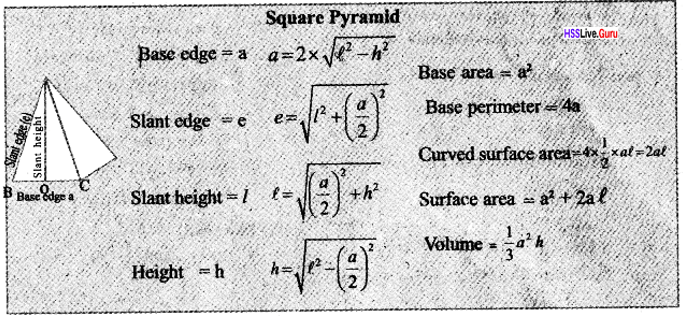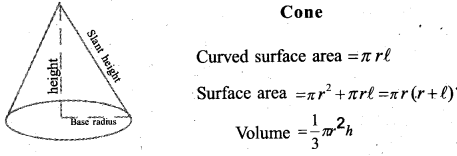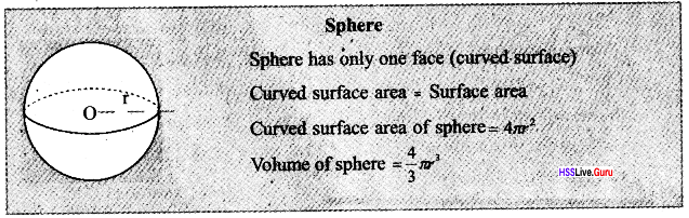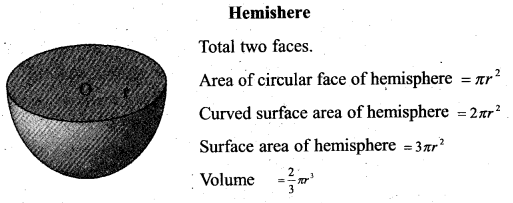# Kerala Syllabus 10th Standard Maths Solutions Chapter 8 Solids

## Kerala State Syllabus 10th Standard Maths Solutions Chapter 8 Solids

### Solids Textbook Questions & Answers

Textbook Page No. 191

Question 1.
A square of side 5 centimetres, and four isosceles triangles!of base 5 centimetres and height 8 centimetres, are to be put together to make a square pyramid. How many square centimetres of paper is needed?
Area of base = 5 × 5 = 25 cm2 Area of one triangle 1/2 × 5 × 8 = 20 cm2 Curved surface area = 4 × 20 5cm = 80 cm2
Paper is needed to make a square pyramid = 25 + 80 = 105 cm2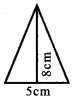Solids in Maths SSLC Question 2. A toy is in the shape of a square pyramid of base edge 16 centimetres and slant height 10 centimetres. What is the total cost of painting 500 such toys, at 80 rupees per square metre?
Surface Area of the toy
= 16 × 16 + 4 × 1/2 × 16 × 10
=256 + 320 = 576 cm2
Surface Area of 500 toys = 500 × 576 = 288000 cm2Kerala Syllabus 10th Standard Maths Question 3. The lateral faces of a square pyramid are equilateral triangles and the length of a base edge is 30 centimetres. What is its surface area?
Lateral faces are equilateral surfaces Surface area
= Base Area + Curved surface area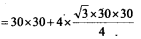= 900 + 900 √3
=900 + 1558.8 = 2458.8 cm2 = 2459 cm2Question 4.
The perimeter of the base of square^»yra- mid is 40 centimetres and the total length of all its edges is 92 centimetres. Calculate its surface area.
Base perimeter = 4a = 40
a=10cm
Total length of edges = 92 cm
Length of total laterals edge = 92 – 40 = 52
Length of one laterals edge = $$\frac { 52 }{ 4 }$$ = 13 cm
Surface area of pyramid = a 2 + 2 al
= 102 + 2 × 10 × 13
= 100 + 260 = 360 cm2

Surface Area of Kerala Question 5. Can we make a square pyramid with the lateral surface area equal to the base area?
Curved surface area = 2al
Area of base = a2
a2=2al
a = 21 ⇒ 1 = a/2
For making a square pyramid first we must determine its base, one side of the lateral will be the base. Other two sides make half of base by reducing the angle. That is angle at apex will be less than 90°.

Textbook Page No. 193

Question 1.
Using a square and four triangles with dimensions as specified in the picture, a pyramid is made.What is the height of this pyramid?
What if the square and triangles are like this?Question 2.
A square pyramid of base edge 10 centimetres and height 12 centimetres is to be made of paper. What should be the dimensions of the triangles?
Base edge of a square pyramid = 10 cm
Let h be the height
Base edge a = 10 cm
Height h= 12 cm
Slant height =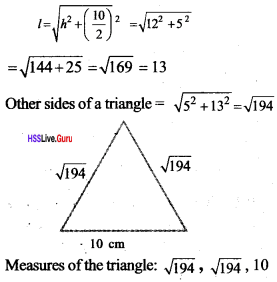Question 3.
Prove that in any square pyramid, the squares of the height, slant height and lateral edge are in arithmetic sequence.
Height = h,
Slant height = l,
Lateral edge = eSolids in Maths Question 4. A square pyramid is to be made with the triangles shown here as a lateral face. What I would be its height? What if the base edge is 40 centimetres instead of 30 centimetres?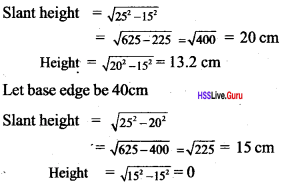It is impossible to make a square pyramid of base edge 40cm.

Textbook Page No. 195

Question 1.
What is the volume of a square pyramid of base edge 10 centimetres and slant height 15 centimetres?
a = 10, l = 15
Volume of pyramid =Question 2.
Two square pyramids have the same volume. The base edge of one is half that of the other. How many times the height of the second pyramid is the height of the first?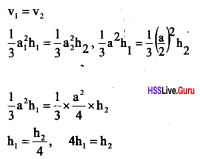The height of the second pyramid is 4 times the height of the first pyramid.

Question 3.
The base edges of two square pyramids are in the ratio 1:2 and their heights in the ratio 1:3. The volume of the first is 180 cubic centimetres. What is the volume of the second?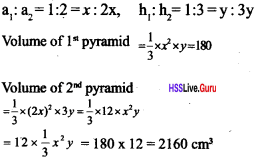Question 4.
All edges of a square pyramid are 18 centimetres. What is its volume?
Length of base edge a = 18 cm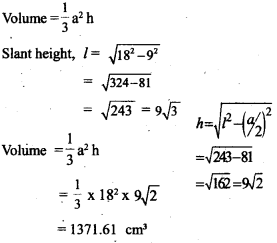Question 5.
The slant height of a square pyramid is 25 centimetres and its surface area is 896 square centimetres. What is its volume?
l = 25 cm
Surface area = 896 cm
a2 + 2al = 896
a2 + 2a × 25 = 896
a2 + 50a – 896 = 0Question 6.
All edges of a square pyramid are of the same length and its height is 12 centimetres. What is its volume?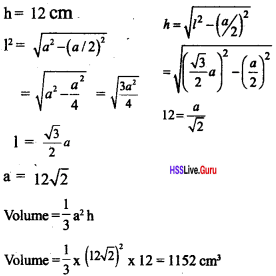Question 7.
What is the surface area of a square pyramid of base perimeter 64 centimetres and volume 1280 cubic centimetres?
Base perimeter 4a = 64
a= 16 cm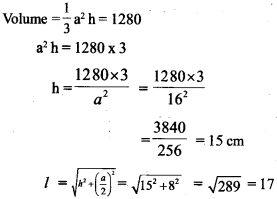Surface area = a2 + 2al
= 162 + 2 × 16 × 17 = 256 + 544 = 800 cm2

Textbook Page No. 198

Question 1.
What are the radius of the base and slant height of a cone made by rolling up a sector of central angle 60° cut out from a circle of radius 10 centimetres?
R = Radius of the circleRadius of cone = 1.66 cm
Radius of circular part = slant height of cone = 10 cm.Question 2.
What is the central angle of the sector to be used to make a cone of base radius 10 centimetres and slant height 25 centimetres?
Central anglejof the sector
(x) = $$\frac { r }{ l }$$ × 360,
r = 10 cm, l = 25 cm
$$=\frac{10}{25} \times 360$$
= 144°

Question 3.
What is the ratio of the base-radius and slant height of a cone made by rolling up a semicircle?
Radius of bigger circle = R
Radius of smaller circle = r
Radius of circular base of the pyramid = r = $$\frac { R }{ 2 }$$
Ratio between radius and slant height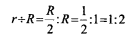Textbook Page No. 199

Question 1.
What is the area of the curved surface of a cone of base radius 12 centimetres and slant height 25 centimetres?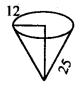r = 12cm l = 25cm
Curved surface area = πrl
π × 12 × 25 = 300π = π × 12 × 25
= 300 × 3.14 = 314 × 3
= 942 cm2

Question 2.
What is the surface area of a cone of base diameter 30 centimetres and height 40 centimetres?
Radius = $$\frac { 30 }{ 2 }$$= 15 cm =r
Height = h = 40 cm
Total surface area = Base area + Curved surface
area = πr2 + πrl= 865 πr = 2718.6 m2

Question 3.
A Conical firework is of. base diameter 10 centimetres and height 12 centimetres, 10000 such fireworks are to be wrapped in colour paper. The price of the colour paper is 2 rupees per square metre. What is the total cost?
r = 5 cm
h =12 cm
t = 13 cm
Total surface area of one firework = Base area + Curved surface area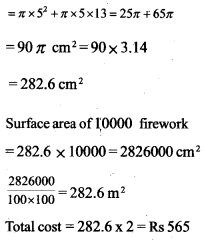Question 4.
Prove that for a cone made by rolling up a semicircle, the area of the curved surface is twice the base area.
Perimeter of base of a cone is equal to half of perimeter of large cone.
Perimeter of base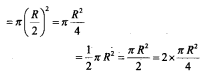= 2 × Base area , that is twice

Textbook Page No. 200

Question 1.
The base radius and height of a cylindrical block of wood are 15 centimetres and 40 centimetres. What is the volume of the largest cone that can be carved out of this?
r = 15cm, h = 40cm
Volume of cone = $$\frac { 1 }{ 3 }$$ πr² h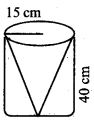= $$\frac { 1 }{ 3 }$$ π × 15 × 15 × 40
= 3000π = 3000 × 3.14
= 314 × 30
= 9420 cm3Question 2.
The base radius and height of a solid metal cylinder are 12 centimetres and 20 centimetres. By melting it and recasting, how many cones of base radius 4 centimetres and height 5 centimetres can be made?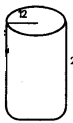No. of cones = Volume of cylinder/Volume of cone Volume of cylinder
= π × 12 × 12 × 20
Volume of cone = $$\frac { 1 }{ 3 }$$ π × 4 × 4 × 5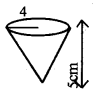Question 3.
A sector of central angle 216° is cut out from a circle of radius 25 centimetres and is rolled up into a cone. What are the base radius and height of the cone? What is its volume?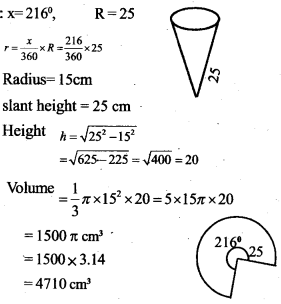Question 4.
The base radii of two cones are in the ratio 3:5 and their heights are in the ratio 2 : 3. What is the ratio of their volumes?Question 5.
Two cones have the same volume and their base radii are in the ratio 4:5. What is the ratio of their heights?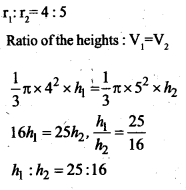Textbook Page No. 203

Question 1.
The surface area of a solid sphere is 120 square centimetres. If it is cut into two halves, what would be the surface area 0f each hemisphere?
Surface area of the solid sphereSurface area of one hemisphere = 3 πr2
3 πr2 = 3 π × $$\frac { 30 }{ π }$$ = 90 cm2

Question 2.
The volumes of two spheres are in the ratio 27 : 64. What is the ratio of their radii? And the ratio of their surface areas?
Ratio of volumesQuestion 3.
base radius and length of a metalder are 4 centimetres and 10 centimetres, If it is melted and recast into spheres of radius 2 centimetres each, how many spheres can be made?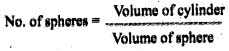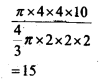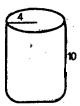Question 4.
A metal sphere of radius 12 centimetres is melted and recast into 27 small spheres. What is the radius of each sphere?
Volume of bigger sphere
$$=\frac{4}{3} \pi \mathrm{R}^{3}=\frac{4}{3} \pi \times 12^{3}$$
If the radius of smaller sphere is ‘r’
Volume of 27 smaller spheres = Volume of the bigger sphereQuestion 5.
From a solid sphere of radius 10 centimetres, a cone of height 16 centimetres is carved out What fraction of the volume of the sphere is the volume of the cone?
Radius of sphere = 10 cm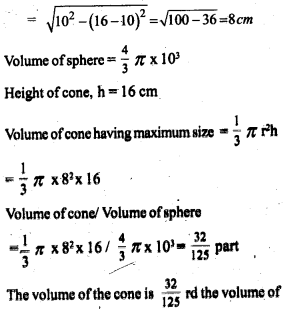Question 6.
The picture shows the dimensions of a petrol tank.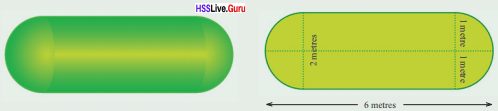How many litres of petrol can it hold?
Length of circular cylinder = 4 m
Height = 4 m
Volume of circular cylinder = π × 12 × 4 = 4π
= 4 × 3.14 = 12.56 cm3
Volume of two hemisphere = Volume of a sphere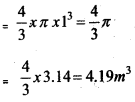Litres of petrol the tank can hold = 12.56 + 4.19 = 16.73 m3 = 16750 litre

Question 7.
A solid sphere is cut into two hemispheres. From one, a square pyramid and from the other a cone, each of maximum possible size are carved out. What is the ratio of their volumes?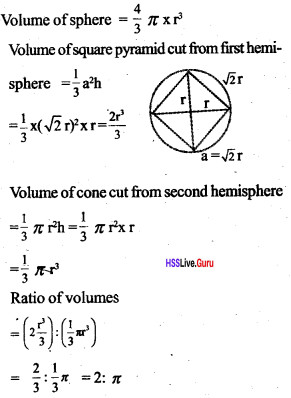### Solids Orukkam Questions & Answers

Worksheet 1

Question 1.
The base edge of a square pyramid is Stem, height 3cm. Calculate slant height and lateral edge
Base edge = 8 cm, height = 3 cm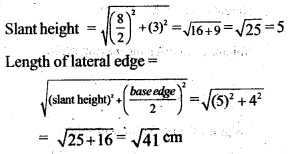Question 2.
Slant height of a square pyramid is 10cm, height 6cm .Calculate total length of the edges.
Slant height =10 cm, height = 6 cm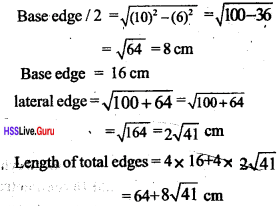Question 3.
The slant height of a square pyramid is 12 cm, lateral edge 13 cm. Calculate height
Slant height = 12 cm
lateral edge = 13 cmQuestion 4.
The length of base edge is 24 cm, slant height 13 cm. Find height and lateral edge
Base edge = 24 cm
Slant height = 13 cm
Height $$=\sqrt{(13)^{2}-(12)^{2}}=\sqrt{25}=5 \mathrm{cm}$$
Length of lateral edge = $$=\sqrt{(13)^{2}-(12)^{2}}=\sqrt{313} \mathrm{cm}$$

Worksheet 2

Question 5.
A sector is folded in such a way as to get a cone. Radius of the sector is 12 cm, central angle 120°.Calculate radius and slant height
Slant height of cone = radius of sector = 12 cm
Radius of cone = $$\frac { 120 }{ 360 }$$ of radius of sector = 12 × $$\frac { 120 }{ 360 }$$ = 4 cm

Question 6.
The central angle of a sector is 90°, radius 16cm, calculate slant height and radius
slant height of cone = 16 cm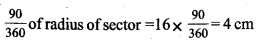Question 7.
Slant height of a cone is 20 cm, radius 10 cm. What should be the radius and central angle of the sector?
Radius of sector = slant height of cone = 20 cm
Central angle of the sector = radius of cone $$\times \frac{360}{R}=10 \times \frac{360}{20}=18^{\circ}$$

Question 8.
Radius of a cone is 4cm, slant height is 5/2 times radius. Calculate the radius and central angle of the sector.
Radius of sector = slant height of cone =Worksheet 3

Question 9.
The base edge of a square pyramid is 6cm, height 4cm, calculate slant height and total surface area.
Length of base edge = 6 cm, height = 4 cm,
slant height = $$\sqrt{(3)^{2}+(4)^{2}}=$$ $$\sqrt{9+16}=\sqrt{25}=5 \mathrm{cm}$$
Total surface area = base area + curved surface area=(6)2 + 2 × 6 × 5 = 36 + 60 = 96 cm2.

Question 10.
The height of a square pyramid is 12cm, slant height 15cm , calculate total surface area and volume
height =12 cm. slant height = 15 cm
$$a=2 \sqrt{15^{2}-12^{2}}$$ = 2 × 9 = 18 cm2
Total surface area = base- area + curved surface area
= (18)2 + 2 × 18 × 15 = 324 + 540 = 864 cm2
Volume = $$\frac{1}{3}(18)^{2} \times 12=1296 \mathrm{cm}^{3}$$

Question 11.
The base perimeter of a square pyramid is 48cm. Slant height is 10cm. Calculate lateral surface area and volume.
Base perimeter = 48cm
baseedge = $$\frac { 48 }{ 4 }$$ = 12 cm, slant height = 10cm
height = $$\sqrt{(10)^{2}-(6)^{2}}=\sqrt{64}=8 \mathrm{cm}$$
Curved siuface area= 2 × 12 × 10 = 240 cm2
Volume = $$\frac { 1 }{ 3 }$$ (12)2 × 8 = 384 cm3

Question 12.
The height of a square pyramid is 15cm, volume 1620cm. Calculate the total surface area.
Volume of square pyramid =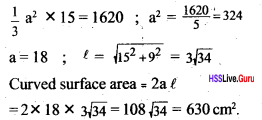Worksheet 4

Question 13.
The base area of a cone is 25 π cm, curved surface area 165 π. Calculate total surface area.
Total surface area of cone = base area + curved surface area
= 25 π +165 π = 190 cm2

Question 14.
Base area of a cone is 81 π, height 12 cm. Calculate volume
Volume of cone = $$\frac { 1 }{ 3 }$$ × base perimeter ×
height = $$\frac { 1 }{ 3 }$$ × 81 π × 12 = 324 π cm3

Question 15.
The height of a cone is 4cm, slant height 5cm. Calculate total surface area
Height of cone = 4 cm
Slant height = 5 cmSurface area = π (3)2 + π x 3 x 5 = 9 π + 15 π =24 π cm2

Question 16.
Radius of a cone is 10cm, volume 3140 cubic centimeter. Calculate total surface area
Radius of cone = 10 cm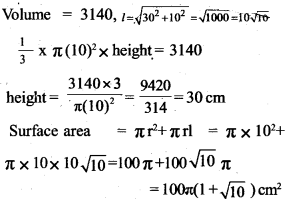Question 17.
Calculate the surface area and volume of a sphere of radius 3 cm.
Surface area of sphere = 4 π (3)2 = 36 π cm2
Volume = $$\frac{4}{3} \pi r^{3}=\frac{4}{3} \times \pi \times(3)^{3}=36 \pi \mathrm{cm}^{3}$$

Workshee 5

Question 18.
Calculate the volume of a sphere of surface area 144 π square centimetre.### Solids SCERT Questions & Answers

Question 19.
The measurements of the lateral surface of a square pyramid are shown in the figure. Calculate die base edge and slant height of die pyramid. [Score: 2, Time: 3 minute]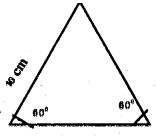Base edge = 10cm (1)
The given figure can be divided into n two right-angled triangles their angles are 30°, 60°, 90°, so ratio of their side will be 1: √3: 2.
2x = 10, x = 5
Slant height = 5 √3 cm (1)3

Question 20.
Is It possible to construct a pyramid of base edge 24 cm and lateral edge 13 cm? Justify [Score: 2, Time: 3 minute]
Since slant height is 5 cm, such a pyramid can’t be constructed. (1)
Slant height should be greater than half of the base edge. $$\sqrt{13^{2}-12^{2}}=5 \mathrm{cm}$$ (1)

Question 21.
Lateral surface of a square pyramid is shown in the Figure. All angles are equalFind the total length of all edges of the square: pyramid. Find the slant height What is the |atio between height and slant height [Score: 4, Time: 5 minutes]
Sum of edges = 8 x 8 = 64 cm (1)
Slant height = 4√3 cm (1)Question 22.
Devikamade a square pyramid having base edge 40cii and height 15cm. Unfortunately, one lateral face got separated from the pyramid. Check which figure given below shows the isosceles triangle that got separated. [Score: 3, Time: 5 minute]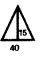Square pyramid can’t be constructed since slant height 1 should be greater than half the base edge. (1)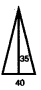If base edge = 40 and slant leight = 35, then height can’t be 15 (1)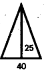Here height of pyramid is 15, so this is the isosceles triangle that got separated (1)

Question 23.
A tent constructed in the form of a square pyramid of base perimeter 80 metres and lateral edge 26 metres
a. Calculate the slant height of the tent
b. Calculate the area of tarpaulin sheet. required to cover the lateral faces of the tent. [Score: 3, Time: 5 minute]
Base perimeter = 80 m, Base edge= 20 m Lateral edge = 26 m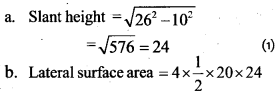= 960 sq.metre (2)

Question 24.
The triangle given in the figure is one lateral face of a square pyramid.
a. Calculate the slant height.
b. Find the lateral surface area of the pyramid, [Score: 3, Time: 4 minutes]Slant height $$=\sqrt{17^{2}-8^{2}}$$
$$=\sqrt{275}=15 \mathrm{cm}$$ (2)

Question 25.
A square pyramid is made from a solid cube having edge 30cm. Calculate the surface area. [Score: 3, Time: 5 minute]
Base edge of the square pyramid = 30 cm
Height = 30cm
Slant height = $$\sqrt{30^{2}+15^{2}}$$
$$=\sqrt{900+225}=\sqrt{1125}=15 \sqrt{5}$$ (1)
Lateral surface area = $$=4 \times \frac{1}{2} \times 15 \sqrt{2} \times 30$$
= 60 x 15√5 = 900√5 sq.cm (1)
Total surface area = 900 + 900√5
= 900(1 + √5) sq.cm (1)

Question 26.
The lateral faces of a square pyramid are equilateral triangles Lateral, edge = 20 cm
a. Calculate the slant height
b. Find its surface area.
C. Find its volume. [Score: 5, Time: 6 minute]
a. Slant height = 10 √3 cm (1)
b. Lateral surface area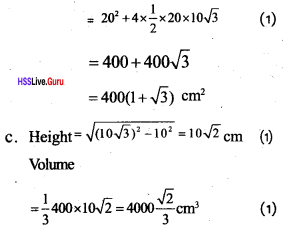Question 27.
Prove that the ratio between the base edge, slant height and height of a square pyrarmid having equal edges is 2: √3 : √2. [Score: 4, Time: 5 minute]
Slant height = √3 a
Height = $$\begin{array}{l}{=\sqrt{(\sqrt{3} a)^{2}-a^{2}}} \\ {=\sqrt{2} a}\end{array}$$
Base edge: Slant height : Height
= 2a: √3a : √2 a = 2: √3 : √2 (1)

Question 28.
The ratio between the base edges of two square pyramids is 1: 2. The heights are also in the same ratio. If the volume of the first pyramid islO cubic centimeters, calculate the volume of the sec ond one. [Score: 3, Time: 4 minute]
v1 : v2 = 1 : 8
v1 = $$\frac { 1 }{ 3 }$$ a2 h
v2 = $$\frac{1}{3}(2 a)^{2} \times 2 h, v_{1}: v_{2}=1: 8$$ (1)
Volume of the second pyramid = 800 cm2 (1)Question 29.
Meera constructed a square pyramid of base edge 10cm and height 6cm Manu made a square pyramid having base edge 5cm and height 4cm. Find the volume of the pyramids and compare the measurements. [Score: 3, Time: 4 minutes]
Volume of Meera’s pyramid = $$\frac { 1 }{ 3 }$$ x Baste edge x height 3
= $$\frac { 1 }{ 3 }$$ x 102 x 6 =200 cm3 (1)
Volume of Manu’s pyramid = $$\frac { 1 }{ 3 }$$ x 52 x 24 = 200cm3
Volumes are equal (1)

Question 30.
The central angle of a sector is 288° If this sector is rolled up to make a cone, find the ratio between the radius and slant height of the cone. [Score :: 4, Time: 5 minutes]
360 x $$\frac { 4 }{ 5 }$$ = 288
∴ Radius of the cone 4 = $$\frac { 4 }{ 5 }$$ x radius of the big circle (1)
∴ If r is the radius of the circle Radius of the
Radius of the cone = $$\frac { 4 }{ 5 }$$ r (1)
But radius of the circle = slant height of cone
i.e., 1 = r (1)
∴Ratio between the radius of the cone and slant height
$$=\frac{4}{5} r: r=\frac{4}{5}: 1=4: 5$$ (1)

Question 31.
The ratio between the radius and slant height of a cone is 2 : 3. Find the central angle of the sector to make the cone. [Score: 3, Time: 4 minutes]
Ratio between the radius and slant height 2 : 3 (1)
Area length of the sector is equal to $$\frac { 2 }{ 3 }$$ part of the circle perimeter. (1)
Central angle of the sector = 360 x $$\frac { 2 }{ 3 }$$ = 240° (1)

Question 32.
The central angle of a circle is divided in the ratio 2 : 3 to form two sectors. Two cones are made by rolling up the two Rectors.
a. Find out the ratio between the base perimeters of the cones.
b. What is the ratio between the curved surface areas. [Score: 3, Time 6 minutes]
a. Central angles are in the ratio 2 : 3 , so, let the perimeter of the two clones which made by rolling up this two sectors be $$\frac { 2 }{ 5 }$$
part and $$\frac { 3 }{ 5 }$$ part of the perimeter of the circle (1)
That is perimeter of each sector be $$2 \pi r \times \frac{2}{5} \text { and } 2 \pi r \times \frac{3}{5}$$
Ratio between base perimeters of cone = $$2 \pi r \times \frac{2}{5}: 2 \pi r \times \frac{3}{5}=2: 3$$
b. Base perimeter of the cones will be $$\frac { 2 }{ 5 }$$ and $$\frac { 3 }{ 5 }$$ parts of the circumference of the 5 circle. (1)
Ratio between the perimeters of die cones = $$\pi r^{2} \times \frac{2}{5}: \pi r^{2} \times \frac{3}{5}=2 ; 3$$ (1)

Question 33.
Find the ratio between the radius and slant height of a cone by roiling up a sector with central angle 120°. If the curved surface area is 108 π, find the radius and slant height of the cone. [Score:5,Time:7minutesv]
Area of the sector with central angle 120° is one-third of the area of the circle. (1)
Curved surface area = 108 π
Ares of die; sector = 108 π,
which is one-third of the area of the circle Area of the circle = 108 π × 3
πr² = 324 π (1)
Radius of the circle r = 18 cm
Slant height = 18 cm (1)
Radius of the cone = 6 cm (1)

Question 34.
A wooden cone is has radius 30crn and height 40crn. Find its slant height. Calculate the cost to paint the face of 10 such cones at the rate of Rs.50/- per square metre. [Score: 5, Time: 7 minutes]
Height =40 cm
Slant height = $$\sqrt{40^{2}+30^{2}}=50$$ (1)
Surface area of the cone = πr² + πrl = π × 302 + π × 30 × 50 (1)
= 900π + 1500π = 2400π (2)
Total cost to paint 10 cones $$=\frac{2400 \pi \times 10 \times 50}{10000}$$
= $$=\frac{2400 \times 3.14 \times 10 \times 50}{10000}=377 \mathrm{Rs}$$ (1)Question 35.
Two cones are made using two sectors of central angles 60° and 120° of a circle. If the radius of the smaller cone is 5cm
a. Calculate the radius and base area of the smaller cone.
b. Find the surface area of the bigger cone. [Score: 5, Time: 8 minutes]
a. Central angle of the small sector = 60°
$$\frac { 1 }{ 6 }$$ part of the area of the circle Base radius of cone formed from above sector = 5 cm (1)
Radius of the circle = 5 × 6 = 30 (1)
Similarly, area of the sector of central angle 120° = $$\frac { 1 }{ 3 }$$ of the area of the circle Base radius ofthe bigger cone = 30 × $$\frac { 1 }{ 3 }$$ = 10 (1)
Base area of the bigger cone = π × 102 = 100× (1)
b. Curved surface area ofthe bigger cone = π × 10 × 30 = 300π (1)
Surface area = 100π + 300π = 400π cm2 (1)

Question 36.
Three solids a square pyramid, a cone and a sphere have been carved out from three solid cubes of the same size. Find the volume of each solid. [Score: 5, Time: 7 minutes]
Volume of square pyramid = $$\frac { 1 }{ 3 }$$ a3 (1)
Volume of coneQuestion 37.
A metal sphere is melted and recasted into a cone. Both have same radii
a. Find the relationship between the height of the cone and the radius of the sphere.
b. Which solid has greater surface area? Justify. [Score: 5, Time: 7 minutes]
a. If r is the radius of the sphere, then its volume = $$\frac { 4 }{ 3 }$$ πr3 (1)
If h is the height of the cone, then its volume = $$\frac{1}{3} \pi r^{2} h=\frac{4}{3} \pi r^{3}$$
h = 4r (1)
Height of pyramid is four times the radius of sphere.
Surface area of the sphere = 4πr²
Slant height of the cone = $$\sqrt{(4 r)^{2}+r^{2}}=\sqrt{17 r^{2}}=\sqrt{17} r$$ (1)
Surface area of the cone = $$\pi r^{2}+\pi r \sqrt{17} r=\pi r^{2}(1+\sqrt{17})$$
b. .Surface area of the cone is greater (1)

Question 38.
A hemisphere and a cone with same radii are attached to get a solid as given in the figure. Radius of the hemisphere is 9 cm. The height of the two solids together is 21 cm.
a. Find the height of the cone.
b. Find the volume of the cone
c. Find the volume of the solids [Score: 4, Time: 6 minutes]
Height of the cone 21 – 9 = 12 cm (1)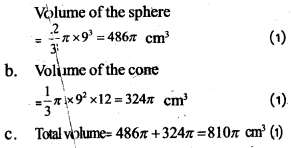### Solids Exam Oriented Questi0ns& Answers

Short Answer Type Questions (Score 2)

Questi0n 39.
Total surface area of a solid hemisphere is 675 π sqcm. Find the curved surface area of the solid hemisphere.
3 πr² = 675π cm2
r2 = 225
The CSA of the solid hemisphere,
CSA = 2πr² = 2π × 225 = 450π cm2

Questi0n 40.
The volume of a solid right circular cone is 4928 cm3. If its height is 24 cm, them find the radius of the cone.
V = 4928 cm3 and h = 24 cmQuesti0n 41.
The figure given below has the total length 20cm height and common diameter 6cm. Find the volume of the figure.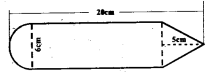volume of solid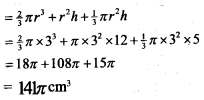Questi0n 42.
The circular plate of radius 12cm is cut out into six sectors having same size. Calculate the slant height and radius of circular cone used to make one sector.
slant height = 12cm
centre angle = $$\frac { 360 }{ 60 }$$ = 60
radius of square pyramid = 12 × $$\frac { 60 }{ 360 }$$ = 2 cmQuesti0n 43.
A circus tent is in the shape of a square pyramid. The area of the base is 1600m2 and its height is 375m then, how much canvas would be needed for this and also find its perimeter?Questi0n 44.
Height of a cone is 40cm. Slant height is 41cm.
a. Find diameter of its base,
b. Find volume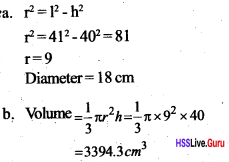Questi0n 45.
Radius and slant height of a solid right circular cone are 35cm and 37 cm respectively. Find the curved surface area and total surface area of the cone.
r= 35 cm
l = 37 cm
CSA = πrI = π(35 x 37)=4070 cm2
TSA = πr (1 + r )
$$=\frac{22}{7} \times 35 \times(37+35)=7920 \mathrm{cm}^{2}$$

Questi0n 46.
Surface area of a wooden sphere is 40 cm2. It is cut into two identical hemispheres. Find
a. The area of the plane surface of one of the hemispheres,
b. Its surface area.
a. Surface area of the sphere = 4 πr²
Here, 4 πr² = 40cm2
πr² = 10cm2
∴ Area of plane surface = πr² = 10cm2
b. Surface area of one piece (hemisphere)
= 3 πr² = 3 x 10 = 30cm2

Short Answer Type Questions (Score 3)

Questi0n 47.
A toy in the shape of a square pyramid has base edge 16 cm and slant height 10 cm. 500 of these are to be painted and the cost is 80 rupees per square meter. What would be the total cost?
Surface area = Curved surface area + base area
4 × 1/2 × 16 × 10+ 162 = 320 + 256 = 576cm
Total surface area of 500 square prism is = 500 × 576 = 288000 cm2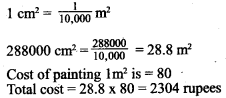Questi0n 48.
The base radius of a circular cone is 9cm and its height is 12cm. What is the radius and central angle of die sector used to make it?
height h =12cm
slant height l = $$\begin{array}{l}{=\sqrt{12^{2}+9^{2}}=\sqrt{144+81}} \\ {=\sqrt{225}=15 \mathrm{cm}}\end{array}$$
radius of the sector = 15cm
centre angle of sector = 360 × $$\frac { 9 }{ 15 }$$ = 216°

Questi0n 49.
The central angle of a sector is 120°. What is the ratio of radius and slant height of a circular cone made by it? What is the radius and slant height of a cone if its curved surface area is 108 π cm2.
The ratio of radius and slant height = $$\frac { 120 }{ 360 }$$ = $$\frac { 1 }{ 3 }$$ = 1 : 3
curved surface area (πrl) =108π
rl=108; r × 3 r = 108
3 × r2 = 108; r2 = 36
radius (r) = 6 cm; slant height (l) = 3r = 3 × 6 = 18cm

Questi0n 50.
For constructing a square pyramid, Rabiya cut of four triangles and a square. Figure given below shows the measures of these triangles and square. Can you make a square pyramid by using these measures? Explain the reason.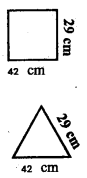Base edge = 42 cm
Slant edge = 29 cm
Slant height = $$\sqrt{29^{2}-\left(\frac{42}{2}\right)^{2}}=\sqrt{29^{2}-21^{2}}$$
$$\sqrt{841-441}=\sqrt{400}=20 \mathrm{cm}$$
Slant height is less than the half of the base edge 21cm so not possible for making a square pyramid

Long Answer Type Questions (Score 4)

Questi0n 51.
A sector shown in the figure is rolled up and made a cone. Find its
a. Slant height
c. Volumea. Slant height = radius of the
sector = 30cm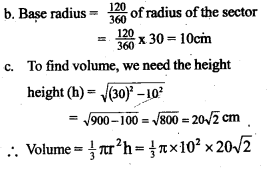$$\frac{2000 \sqrt{2 x}}{3} \mathrm{cm}^{3}$$Questi0n 52.
Paddy is filled in a cylindrical shaped vessel. Then it has the following shape. How many litres of paddy does it contain?Questi0n 53.
A petrol tank is in the shape of a cylinder with hemisphere of the same radius as the base of the cylinder attached to both ends. If the total length of the tank is 5 meters and the base radius of the cylinder is 1 metre, how many litres of petrol can it hold?
Volume of cylinder = πr²h = 3πcm3
r=1, h=3
Volume of hemisphere = 2/3 πr3Long Answer Type Questions (Score 5)

Questi0n 54.
A toy is in the shape of a hemisphere attached to one end of the cone Total height of the toy is 14.5cm, and common diameter is 7cm.
a. Draw a rough figure based on this fact,
b. Find the volume of the toy.Questi0n 55.
Prove that:
i. ∠BAT = ∠BPA
ii. ∠BAS = ∠AQB